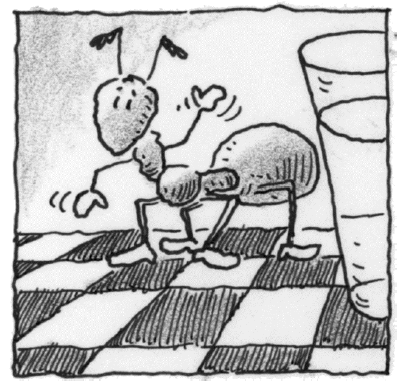### Home > CALC3RD > Chapter Ch5 > Lesson 5.2.1 > Problem5-54

5-54.

There is an ant moving along the edge of your table. Its position from the center of one edge of the table is given by the equation $x(t) = (t – 1)(t – 4)^4$. When $x\left(t\right)$ is increasing, the ant is moving forward.

1. What is $\frac { d x } { d t }$?

Use the Product and Chain Rules.

2. If $x\left(t\right)$ represents how many millimeters the ant is from the center of the table’s edge after $t$ seconds, what are the units for $\frac { d x } { d t }$? What information does this derivative give about the ant?

$\text{Recall that }\frac{dx}{dt} \text{ means }\lim \limits_{\Delta t\rightarrow 0}\frac{\Delta x}{\Delta t}.$

What does $x$ and $t$ stand for?

3. What are the units for $\frac { d ^ { 2 } x } { d t ^ { 2 } }$? What information does this second derivative give about the ant?

Refer to the hint in part (b).

4. How often does the ant stop and change direction? At what time(s)? Be sure to find and confirm your answer using both calculus techniques and a graph.

Analytic method: The ant will change directions when $x^\prime\left(t\right)$ changes sign.
Graphical method: The ant will change directions when the derivative graph CROSSES the $x$-axis.

5. At time $t = 3$, is the ant moving forwards or backwards? Justify your answer using calculus.

When velocity is positive, the ant is moving forward. When velocity is negative, the ant is moving backwards.